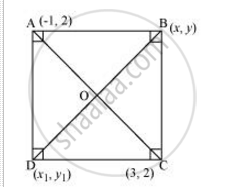Share

# The two opposite vertices of a square are (− 1, 2) and (3, 2). Find the coordinates of the other two vertices. - CBSE Class 10 - Mathematics

#### Question

The two opposite vertices of a square are (− 1, 2) and (3, 2). Find the coordinates of the other two vertices.

#### SolutionLet ABCD be a square having (−1, 2) and (3, 2) as vertices A and C respectively. Let (xy), (x1y1) be the coordinate of vertex B and D respectively.

We know that the sides of a square are equal to each other.

∴ AB = BC

=>sqrt((x+1)^2 + (y-2)^2) = sqrt((x-3)^2 + (y-2)^2)

=>x2 + 2x + 1 + y2 -4y + 4 = x2 + 9  -6x + y2 + 4 - 4y

⇒ 8x = 8

⇒ x = 1

We know that in a square, all interior angles are of 90°.

In ΔABC,

AB2 + BC2 = AC2

=> (sqrt(((1+1)^2)+(y-2)^2))^2 + (sqrt(((1-3)^2)+(y-2)^2))^2 = (sqrt((3+1)^2+(2-2)^2))^2

⇒ 4 + y2 + 4 − 4y + 4 + y2 − 4y + 4 =16

⇒ 2y2 + 16 − 8 y =16

⇒ 2y2 − 8 y = 0

⇒ y (− 4) = 0

⇒ y = 0 or 4

We know that in a square, the diagonals are of equal length and bisect each other at 90°. Let O be the mid-point of AC. Therefore, it will also be the mid-point of BD

Coordinate of point O = ((-1+3)/2, (2+2)/2)

((1+x_1)/2, (y+ y_1)/2) = (1,2)

(1+x_1)/2 = 1

1+x1=2

x1 =1

and

 (y + y_1)/2 = 2

⇒ y + y1 = 4

If y = 0,

y1 = 4

If y = 4,

y1 = 0

Therefore, the required coordinates are (1, 0) and (1, 4).

Is there an error in this question or solution?

#### APPEARS IN

NCERT Solution for Mathematics Textbook for Class 10 (2019 to Current)
Chapter 7: Coordinate Geometry
Ex. 7.40 | Q: 4 | Page no. 171

#### Video TutorialsVIEW ALL 

Solution The two opposite vertices of a square are (− 1, 2) and (3, 2). Find the coordinates of the other two vertices. Concept: Area of a Triangle.
S